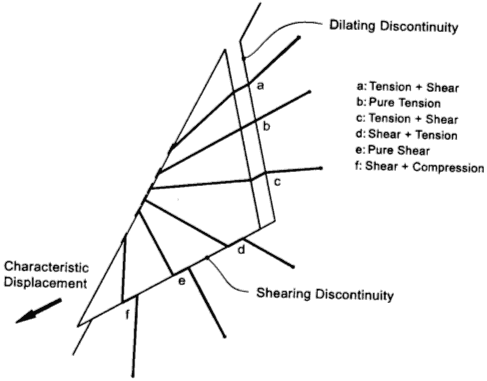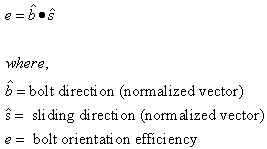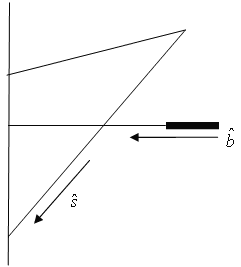The Rocscience International Conference 2021 Proceedings are now available. Read Now

Bolt Orientation Efficiency

Bolts that intersect a wedge in RocPlane can deform in a number of different ways, as shown in the figure below. As a result, the mechanism of failure of a bolt can be quite complicated. In order to simplify the behavior for the purpose of including support in a limit equilibrium wedge analysis program such as RocPlane, an empirical reinforcement efficiency factor can be used.Possible bolt deformation modes [Windsor, 1996]

The theoretical Tensile Capacity of a bolt at any point along its length is determined from the Bolt Force Diagram and the point at which a wedge plane intersects the bolt. This assumes that a bolt is in pure tension. However, pure tension occurs only if the bolt orientation is aligned exactly with the sliding direction or direction of movement of the wedge (see b: Pure Tension in the figure above).

If a bolt is not in pure tension but also shear, the effective Tensile Capacity of the bolt is reduced. The amount of reduction is given by the Bolt Orientation Efficiency factor, which may range between zero and one.

• If the Bolt Orientation Efficiency factor is zero, the bolt has no effect and zero force is placed into the equilibrium equations of the wedge.
• Conversely, if the Bolt Orientation Efficiency factor is one, the bolt is in pure tension and the entire capacity is added as a force in the direction of the bolt in the equilibrium equations of the wedge.

NOTE: The Bolt Orientation Efficiency factor, if used, is ONLY applied to the Tensile Capacity of a bolt as determined from the Bolt Force Diagram. It is NOT applied to the Shear Capacity of a bolt, if the Use Shear Strength option is being used. For an overall summary of the bolt support force implementation in RocPlane, see Bolt Support Force in RocPlane.

Calculation of Bolt Orientation Efficiency Factor

The Bolt Orientation Efficiency factor is calculated by relating the direction of sliding or movement of the wedge to the orientation of the bolt. In RocPlane, there are two different calculation methods.

COSINE TENSION / SHEAR

This is the default method in RocPlane. With reference to the figure below, the efficiency is calculated as:NOTE; This applies to bolt deformation modes A, B, C, D (see the figure above). For bolt deformation modes E (pure shear) or F (compression and shear), the Bolt Orientation Efficiency factor = 0.Bolt orientation and sliding direction used for Bolt Efficiency calculation

LINEAR TENSION / SHEAR

With the Linear Tension / Shear method, the efficiency is calculated as:NOTE: This applies to bolt deformation modes A, B, C, D (see the first figure above). For bolt deformation modes E (pure shear) or F (compression and shear), the Bolt Orientation Efficiency factor = 0.

Bolt Orientation Efficiency Off

The Bolt Orientation Efficiency option can be turned off. To turn off the option, deselect the Use Bolt Orientation Efficiency check box in the Bolt Properties dialog.

If Bolt Orientation Efficiency is OFF, then the Bolt Orientation Efficiency factor = 1, regardless of the bolt deformation mode (A,B,C,D,E,F in the first figure above).

Bolt Efficiency and Shear Strength

Finally, note that Bolt Orientation Efficiency is NOT used in conjunction with the Bolt Shear Strength option. If you have selected the Use Bolt Shear Strength option and the bolt deformation mode is D, E or F so that the Bolt Shear Strength is applied, the Bolt Orientation Efficiency factor DOES NOT multiply the Shear Strength. Bolt Orientation Efficiency is only used as a factor for the Tensile Capacity determined from the Bolt Force Diagram.